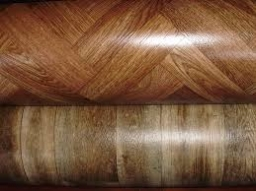# Rectangular 7578

My aunt paid CZK 2,592 for linoleum in a 15 meters long rectangular hallway. How much did 1m of linoleum cost? The following month, linoleum was discounted by a third. How many crowns could the aunt save if she bought it a month later?

x =  172.8 Kc
u =  864 Kc

### Step-by-step explanation:Did you find an error or inaccuracy? Feel free to write us. Thank you!

Tips for related online calculators
Need help calculating sum, simplifying, or multiplying fractions? Try our fraction calculator.
Do you want to convert length units?